• 2022-03-13 14:12:10

## 描述

请补全JavaScript代码，要求返回一个长度为参数值并且每一项值都为参数值的数组。
注意：
1. 请勿直接使用for/while

<!DOCTYPE html>
<html>
<meta charset=utf-8>
<body>

<script type="text/javascript">
const _createArray = (number) => {
//1、通过new Array函数构造实例的时候带入参数，可以生成该参数长度的空数组
//2、通过Array.fill函数可以将数组的每一项都改编为参数值
//3、或Array.from函数接收两个参数即可，第一个参数为数组或对象，都表示返回数组的长度
//当参数为数组时它确定了返回的新数组长度，当参数为对象时，需要添加“length”属性表明数组长度
//4、第二个参数为一个函数，即第一个数组参数中的每一项都调用该函数
return Array(number).fill(number);
}

//第二种方法
const _createArray = (number) =>{
return Array(number).from({length:number},()=>number)
}
</script>
</body>
</html>javascript
更多相关内容
• 创建numpy.array，是使用numpy这个核武器的基础，本文尽量汇总常用创建numpy.array方法array函数>>> import numpy as np>>> a = np.array([1,2,3,4,5])>>> aarray([1, 2, 3, 4, 5])&...

创建numpy.array，是使用numpy这个核武器的基础，本文尽量汇总常用创建numpy.array的方法。

array函数

>>> import numpy as np

>>> a = np.array([1,2,3,4,5])

>>> a

array([1, 2, 3, 4, 5])

>>> a.shape

(5,)

>>> a.ndim

1

>>> a.dtype

dtype('int32')

>>>

>>> b = np.array((1,2,3,4,5.0))

>>> b

array([1., 2., 3., 4., 5.])

>>> b.shape

(5,)

>>> b.ndim

1

>>> b.dtype

dtype('float64')

a用list创建，b用tuple创建，都一样。注意创建b时，tuple中有一个float，这时numpy将所有数据都转换成了它自己的float64类型。numpy.array中不允许有不同类型的数据同时存在。

我们还可以通过array函数创建多维数组：

>>> c = np.array(([1,2,3],[4,5,6],[7,8,9]))

>>> c

array([[1, 2, 3],

[4, 5, 6],

[7, 8, 9]])

>>> c.shape

(3, 3)

>>> c.ndim

2

>>>

>>> d = np.array([[1,2,3],[4,5,6],[7,8,9.0]])

>>> d.dtype

dtype('float64')

>>> d

array([[1., 2., 3.],

[4., 5., 6.],

[7., 8., 9.]])

创建array时，可以指定dtype：

>>> e = np.array((1,2,3), dtype=np.int64)

>>> e

array([1, 2, 3], dtype=int64)

>>>

>>> f = np.array((1,2,3), dtype=np.float16)

>>> f

array([1., 2., 3.], dtype=float16)

zeros函数

用zeros函数创建全0的array：

>>> a = np.zeros((2,3))

>>> a

array([[0., 0., 0.],

[0., 0., 0.]])

>>>

>>> b = np.zeros((3,5), dtype=np.int8)

>>> b

array([[0, 0, 0, 0, 0],

[0, 0, 0, 0, 0],

[0, 0, 0, 0, 0]], dtype=int8)

zeros_like函数

创建一个全0的，跟输入的array的shape一样的：

>>> a = np.array([[1,2,3],[4,5,6]])

>>> a

array([[1, 2, 3],

[4, 5, 6]])

>>> b = np.zeros_like(a)

>>> b

array([[0, 0, 0],

[0, 0, 0]])

>>> a.shape

(2, 3)

>>> b.shape

(2, 3)

>>> c = np.zeros_like(a, dtype=np.uint8)

>>> c

array([[0, 0, 0],

[0, 0, 0]], dtype=uint8)

>>> c.shape

(2, 3)

ones函数

跟zeros函数一样，创建全1的numpy.array，代码略。

ones_like函数

跟zeros_like函数一样，全1而已，注意这些函数都可以指定dtype。

empty函数

跟zeros和ones一样，不一样的地方在于，empty函数不对数据做初始化，即所有的内部数据都是随机的，看内存什么样子。有的时候使用empty函数，不做初始化，还可以进一步提高计算速度。

>>> a = np.empty((5,5))

>>> a

array([[0.00000000e+000, 2.30226697e-307, 2.30240278e-307,

2.30259291e-307, 2.30275588e-307],

[2.13425923e-307, 2.13439504e-307, 2.13453085e-307,

2.13473456e-307, 2.13493827e-307],

[2.13504692e-307, 2.13527779e-307, 2.13554940e-307,

2.13572595e-307, 2.13591609e-307],

[2.51437149e-307, 2.51456162e-307, 2.51476533e-307,

2.51500978e-307, 2.51517275e-307],

[2.51537647e-307, 2.51562092e-307, 2.51582463e-307,

2.51598760e-307, 2.28647257e-317]])

empty_like函数

跟其它*_like函数一样，也跟自己的empty一样，不做初始化。代码略。

arange函数

a range，一个范围，就像python内置的range一样：

>>> a = np.arange(10)

>>> a

array([0, 1, 2, 3, 4, 5, 6, 7, 8, 9])

>>> b = np.arange(0,100,5)

>>> b

array([ 0, 5, 10, 15, 20, 25, 30, 35, 40, 45, 50, 55, 60, 65, 70, 75, 80,

85, 90, 95])

>>> c = np.arange(20).reshape(5,4)

>>> c

array([[ 0, 1, 2, 3],

[ 4, 5, 6, 7],

[ 8, 9, 10, 11],

[12, 13, 14, 15],

[16, 17, 18, 19]])

>>> c.shape

(5, 4)

>>>

>>> d = np.arange(0,1,0.01).reshape(10,10)

>>> d

array([[0. , 0.01, 0.02, 0.03, 0.04, 0.05, 0.06, 0.07, 0.08, 0.09],

[0.1 , 0.11, 0.12, 0.13, 0.14, 0.15, 0.16, 0.17, 0.18, 0.19],

[0.2 , 0.21, 0.22, 0.23, 0.24, 0.25, 0.26, 0.27, 0.28, 0.29],

[0.3 , 0.31, 0.32, 0.33, 0.34, 0.35, 0.36, 0.37, 0.38, 0.39],

[0.4 , 0.41, 0.42, 0.43, 0.44, 0.45, 0.46, 0.47, 0.48, 0.49],

[0.5 , 0.51, 0.52, 0.53, 0.54, 0.55, 0.56, 0.57, 0.58, 0.59],

[0.6 , 0.61, 0.62, 0.63, 0.64, 0.65, 0.66, 0.67, 0.68, 0.69],

[0.7 , 0.71, 0.72, 0.73, 0.74, 0.75, 0.76, 0.77, 0.78, 0.79],

[0.8 , 0.81, 0.82, 0.83, 0.84, 0.85, 0.86, 0.87, 0.88, 0.89],

[0.9 , 0.91, 0.92, 0.93, 0.94, 0.95, 0.96, 0.97, 0.98, 0.99]])

跟python内置的range不一样的地方是，step可以是小数，不过一般不建议这样用，要产生均匀间隔的数据，建议使用下面这个函数：linspace

linspace函数

设置起点和终点，设置一共取多少个数：

>>> a = np.linspace(0,1,100).reshape(10,10)

>>> a

array([[0. , 0.01010101, 0.02020202, 0.03030303, 0.04040404,

0.05050505, 0.06060606, 0.07070707, 0.08080808, 0.09090909],

[0.1010101 , 0.11111111, 0.12121212, 0.13131313, 0.14141414,

0.15151515, 0.16161616, 0.17171717, 0.18181818, 0.19191919],

[0.2020202 , 0.21212121, 0.22222222, 0.23232323, 0.24242424,

0.25252525, 0.26262626, 0.27272727, 0.28282828, 0.29292929],

[0.3030303 , 0.31313131, 0.32323232, 0.33333333, 0.34343434,

0.35353535, 0.36363636, 0.37373737, 0.38383838, 0.39393939],

[0.4040404 , 0.41414141, 0.42424242, 0.43434343, 0.44444444,

0.45454545, 0.46464646, 0.47474747, 0.48484848, 0.49494949],

[0.50505051, 0.51515152, 0.52525253, 0.53535354, 0.54545455,

0.55555556, 0.56565657, 0.57575758, 0.58585859, 0.5959596 ],

[0.60606061, 0.61616162, 0.62626263, 0.63636364, 0.64646465,

0.65656566, 0.66666667, 0.67676768, 0.68686869, 0.6969697 ],

[0.70707071, 0.71717172, 0.72727273, 0.73737374, 0.74747475,

0.75757576, 0.76767677, 0.77777778, 0.78787879, 0.7979798 ],

[0.80808081, 0.81818182, 0.82828283, 0.83838384, 0.84848485,

0.85858586, 0.86868687, 0.87878788, 0.88888889, 0.8989899 ],

[0.90909091, 0.91919192, 0.92929293, 0.93939394, 0.94949495,

0.95959596, 0.96969697, 0.97979798, 0.98989899, 1. ]])

从0到1，100个数。

reshape函数

顾名思义吧，就是一个数组重新变换一下形状，变成多维的。上面的代码已经有很多地方在直接使用reshape函数了，请参考。

再给一个用reshape将一维转四维的示例：

>>> a = np.linspace(0,1,100).reshape(2,5,2,5)

>>> a

array([[[[0. , 0.01010101, 0.02020202, 0.03030303, 0.04040404],

[0.05050505, 0.06060606, 0.07070707, 0.08080808, 0.09090909]],

[[0.1010101 , 0.11111111, 0.12121212, 0.13131313, 0.14141414],

[0.15151515, 0.16161616, 0.17171717, 0.18181818, 0.19191919]],

[[0.2020202 , 0.21212121, 0.22222222, 0.23232323, 0.24242424],

[0.25252525, 0.26262626, 0.27272727, 0.28282828, 0.29292929]],

[[0.3030303 , 0.31313131, 0.32323232, 0.33333333, 0.34343434],

[0.35353535, 0.36363636, 0.37373737, 0.38383838, 0.39393939]],

[[0.4040404 , 0.41414141, 0.42424242, 0.43434343, 0.44444444],

[0.45454545, 0.46464646, 0.47474747, 0.48484848, 0.49494949]]],

[[[0.50505051, 0.51515152, 0.52525253, 0.53535354, 0.54545455],

[0.55555556, 0.56565657, 0.57575758, 0.58585859, 0.5959596 ]],

[[0.60606061, 0.61616162, 0.62626263, 0.63636364, 0.64646465],

[0.65656566, 0.66666667, 0.67676768, 0.68686869, 0.6969697 ]],

[[0.70707071, 0.71717172, 0.72727273, 0.73737374, 0.74747475],

[0.75757576, 0.76767677, 0.77777778, 0.78787879, 0.7979798 ]],

[[0.80808081, 0.81818182, 0.82828283, 0.83838384, 0.84848485],

[0.85858586, 0.86868687, 0.87878788, 0.88888889, 0.8989899 ]],

[[0.90909091, 0.91919192, 0.92929293, 0.93939394, 0.94949495],

[0.95959596, 0.96969697, 0.97979798, 0.98989899, 1. ]]]])

eye函数

eye函数应该是取I的谐音，用于创建单位矩阵(Identity matrix)：

>>> a = np.eye(5)

>>> a

array([[1., 0., 0., 0., 0.],

[0., 1., 0., 0., 0.],

[0., 0., 1., 0., 0.],

[0., 0., 0., 1., 0.],

[0., 0., 0., 0., 1.]])

>>>

>>> a = np.eye(7)

>>> a

array([[1., 0., 0., 0., 0., 0., 0.],

[0., 1., 0., 0., 0., 0., 0.],

[0., 0., 1., 0., 0., 0., 0.],

[0., 0., 0., 1., 0., 0., 0.],

[0., 0., 0., 0., 1., 0., 0.],

[0., 0., 0., 0., 0., 1., 0.],

[0., 0., 0., 0., 0., 0., 1.]])

>>>

>>> a = np.eye(7, dtype=np.uint8)

>>> a

array([[1, 0, 0, 0, 0, 0, 0],

[0, 1, 0, 0, 0, 0, 0],

[0, 0, 1, 0, 0, 0, 0],

[0, 0, 0, 1, 0, 0, 0],

[0, 0, 0, 0, 1, 0, 0],

[0, 0, 0, 0, 0, 1, 0],

[0, 0, 0, 0, 0, 0, 1]], dtype=uint8)

full函数

用同一个固定的数字来填满array中所有的空隙，zeros是用0填，ones是用1填，empty是不填，随机。

>>> a = np.full((3,4), 8)

>>> a

array([[8, 8, 8, 8],

[8, 8, 8, 8],

[8, 8, 8, 8]])

>>> a

array([[8, 8, 8, 8],

[8, 8, 8, 8],

[8, 8, 8, 8]])

>>>

>>> a = np.full((3,4), 8, dtype=np.float64)

>>> a

array([[8., 8., 8., 8.],

[8., 8., 8., 8.],

[8., 8., 8., 8.]])

>>> a = np.full((3,4,5), 8, dtype=np.float64)

>>> a

array([[[8., 8., 8., 8., 8.],

[8., 8., 8., 8., 8.],

[8., 8., 8., 8., 8.],

[8., 8., 8., 8., 8.]],

[[8., 8., 8., 8., 8.],

[8., 8., 8., 8., 8.],

[8., 8., 8., 8., 8.],

[8., 8., 8., 8., 8.]],

[[8., 8., 8., 8., 8.],

[8., 8., 8., 8., 8.],

[8., 8., 8., 8., 8.],

[8., 8., 8., 8., 8.]]])

-- EOF --

展开全文• 文章目录 创建数据 array本身的属性 创建array方法 生成随机数的np.random模块构建 array本身支持的大量操作和函数   Numerical Python(Numpy)是一个开源的Python科学计算库，使用Numpy可以方便的使用数组、矩阵...

### 文章目录

Numerical Python(Numpy)是一个开源的Python科学计算库，使用Numpy可以方便的使用数组、矩阵进行计算，包含线性代数、傅里叶变换、随机数生成等大量函数。

与原生的Python实现相比，使用Numpy是直接以数组、矩阵为粒度计算，并且支持大量的数学函数，而Python需要用for循环从底层实现。并且Numpy的数组存储效率和输入输出计算性能，比Python使用List或者嵌套List好很多。

Numpy的数据存储和Python原生的List是不一样的，Numpy的大部分代码都是C语言实现的，这也是Numpy代码更高效的原因。NumpyarrayPythonList的一个区别，Numpyarray的元素必须都是同一种数据类型，比如都是数字int类型，这也是Numpy高性能的一个原因。

## 创建数据

import numpy as np
x = np.array([1,2,3,4,5]) # 创建一个一维数组

import numpy as np
x = np.array([[1,2,3,4,5],
[2,3,4,5,6]]) # 创建一个二维数组


## array本身的属性

• shape：返回一个元组，表示array的维度
• ndim：一个数字，表示array的维度的数目
• size：一个数字，表示array中所有数据元素的数目
• dtype：array中元素的数据类型
import numpy as np
x = np.array([[1,2,3,4,5],
[2,3,4,5,6]])
print(x.shape) # 输出：(2, 5)

import numpy as np
x = np.array([[1,2,3,4,5],
[2,3,4,5,6]])
print(x.ndim) # 输出 2

import numpy as np
x = np.array([[1,2,3,4,5],
[2,3,4,5,6]])
print(x.size) # 输出 10

import numpy as np
x = np.array([[1,2,3,4,5],
[2,3,4,5,6]])
print(x.dtype) # 输出 int64


## 创建array的方法

从Python的列表List和嵌套列表创建array。使用预定函数arangeones/ones_likezeros/zeros_likeempty/empty_likfull/full_likeeye等函数创建

1. 使用arange创建数字序列

语法arange(start, stop, step, dtype=None)

import numpy as np
x = np.arange(2, 10, 2)
print(x) # 输出 [2 4 6 8]

1. 使用ones创建全是1的数组

语法np.ones(shape, dtype=None, order='C')；shape : int or tuple of ints Shape of the new array, e.g., (2, 3) or 2。

import numpy as np
x = np.ones([2, 3])
# 输出： [[1. 1. 1.]
#        [1. 1. 1.]]

1. 使用ones_like创建形状相同的数组

语法ones_like(a, dtype=float, order='C')

import numpy as np
x = np.arange(2, 10, 2)
y = np.ones_like(x) # 输出：[1 1 1 1]

1. 使用zeros创建全是0的数组

语法np.zeros(shape, dtype=None, order='C')

import numpy as np
x = np.zeros(5) # [0. 0. 0. 0. 0.]

import numpy as np
x = np.zeros([2,3])
# 输出： [[0. 0. 0.]
#        [0. 0. 0.]]

1. 使用zeros_like创建形状相同的数组

语法np.zeros_like(a, dtype=None)

import numpy as np
x = np.arange(2, 10, 2)
y = np.zeros_like(x) # 输出：[0 0 0 0]

1. 使用empty创建全是0的数组

语法empty(shape, dtype=float, order='C')；注意：数据是未初始化的，里面的值可能是随机值不要用：

import numpy as np
x = np.empty(2) # 输出：[-5.73021895e-300  6.94067723e-310]

import numpy as np
x = np.empty((1,2)) # 输出 [[-5.73021895e-300  6.90355282e-310]]

1. 使用empty_like创建形状相同的数组

语法empty_like(prototype, dtype=None)

import numpy as np
x = np.empty((1,2))
y = np.empty_like(x) # 输出 [[-5.73021895e-300  6.90023394e-310]]

1. 使用full创建指定值的数组

语法np.full(shape, fill_value, dtype=None, order='C')

import numpy as np
x = np.full(2, 10) # 输出：[10 10]

1. 使用full_like创建形状相同的数组

语法np.full_like(a, fill_value, dtype=None)

import numpy as np
x = np.full(2, 10)
y = np.full_like(x, 20) # 输出：[20 20]


## 生成随机数的np.random模块构建

1. 使用random模块生成随机数的数组
import numpy as np
x = np.random.randn(2) # 输出：[-0.83420278 -0.74489849]

import numpy as np
x = np.random.randn(2,2,2)


输出：

[[[ 1.57009273  2.7144515 ]
[-0.63920122 -0.69983059]]

[[ 0.54429723 -0.02818363]
[ 0.36522726  1.71868176]]]


## array本身支持的大量操作和函数

1. 直接逐元素的加减乘除等算数操作
2. 更好用的面向多维的数组索引
3. sum/mean等聚合函数
4. 线性代数函数，比如求解逆矩阵、求解方程组
import numpy as np
x = np.arange(10).reshape(2,5)


输出：

[[0 1 2 3 4]
[5 6 7 8 9]]


通过广播机制实现数组中每个元素加1：

import numpy as np
x = np.arange(10).reshape(2,5)
print(x+1)


输出：

[[ 1  2  3  4  5]
[ 6  7  8  9 10]]


对数组中每个元素做个运算：

import numpy as np
x = np.arange(10).reshape(2,5)
print(np.sin(x))


输出：

[[ 0.          0.84147098  0.90929743  0.14112001 -0.7568025 ]
[-0.95892427 -0.2794155   0.6569866   0.98935825  0.41211849]]

import numpy as np
x = np.arange(10).reshape(2,5)
x1 = np.arange(10).reshape(2,5)
print(x*x1)


输出：

[[ 0  1  4  9 16]
[25 36 49 64 81]]

展开全文• 创建array方法3.array本身支持的大量操作和函数三、array的基本操作1.使用python的list和嵌套list创建一维的array和二维的array2.数组array的属性3.创建array的便捷函数<1>.使用arange创建数字序列<2>...

提示：以下是本篇文章正文内容，下面案例可供参考

# 一.Numpy的认知

## 1.Numpy是什么

1.一个开源的Python科学计算库
2.使用Numpy可以方便的使用数组，矩阵进行计算
3.包含线性代数，傅里叶变换，随机数生成等大量函数


## 2.Numpy的优点

代码更简洁：Numpy直接以数组，矩阵为粒度（一个单元）计算并且支持大量的数学函数，而python需要用for循环从底层实现
性能更高效：Numpy的数组存储效率和输入输出计算性能，比python使用list或者嵌套list好很多
注：（Numpy的数据存储和python原生的list是不一样的）
注：Numpy的大部分代码都是c语言实现的，这是Numpy比纯python代码高效的原因


Numpy是Python各种数据科学类库的基础库
如果不会Numpy，那么这些库的深入理解都会遇到障碍

# 二、array的基本知识

## 1.array本身的属性

shape：返回一个元组，表示array的维度
ndim：一个数字，表示array的维度的数目
size：一个数字，表示array元素的个数
dtpye：array中元素的数据类型


## 2.创建array的方法

1.从python的列表list和嵌套列表创建array
2.使用预定函数arrange，ones/ones_like,zeros/zeros_like,full/full_like,eye等函数创建
3.生成随机数的np.random模块构建


## 3.array本身支持的大量操作和函数

1.直接逐元素的加减乘除等算术操作
2.更好用的面向多维的数组索引
3.求sum/mean的等聚合函数
4.线性代数函数，比如求解逆矩阵，求解方程组


# 三、array的基本操作

导入模块：

import numpy as np


## 1.使用python的list和嵌套list创建一维的array和二维的array

# 创建一个一维的数组，也就是python的单元素list
x=np.array([1,2,3,4,5,6,7,8])
# 创建一个二维的数组，也就是python的嵌套list
X=np.array([[1,2,3,4],[5,6,7,8]])
print("x=",x)
print("X=",X)


运行结果：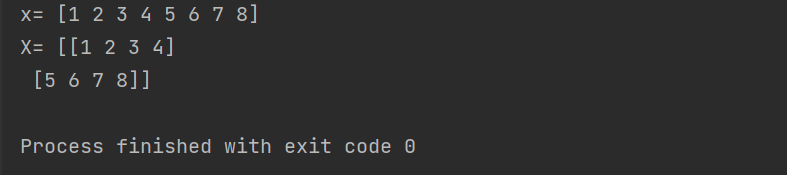## 2.数组array的属性

print(x.shape)#返回一个元组，表示维度和元素个数
print(X.shape)
print(x.ndim)#返回一个值，表示维度
print(X.ndim)
print(x.size)#返回一个值，表示数组中所有元素的个数
print(X.size)
print(x.dtype)#返回一个值，表示数组内元素的数据类型
print(X.dtype)


运行结果：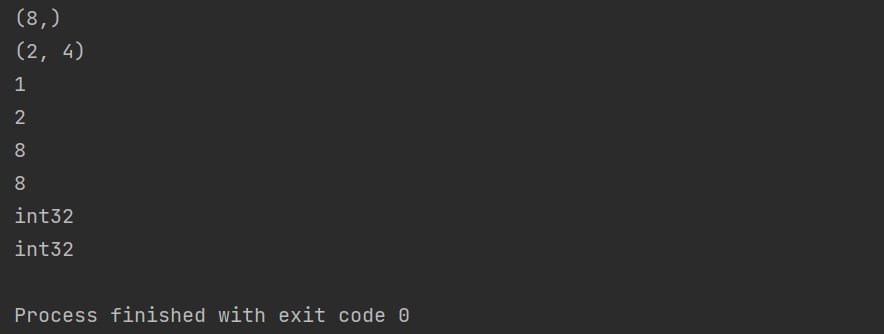## 3.创建array的便捷函数

### <1>.使用arange创建数字序列

arange([起始值（取得到）],[终止值（取不到）],[步长]，dtype=None)

print(np.arange(10))#创建一个0-10的一维数组（取不到10）
print(np.arange(2,10,2))#创建一个2-10，步长为2的一维数组（取不到10）


运行结果：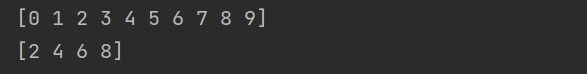### <2>使用ones创建单位数组

np.ones(shape,dtype=None,order=‘C’)
shape:int or tuple of ints Shape of the new array . eg:(2,3) or 2
dtype：数据类型，默认的是自己从输入的数据自动获得
order：有"C"和"F"两个选项,分别代表，行优先和列优先，在计算机内存中的存储元素的顺序

print(np.ones(10))#创建一个一行十列的单位数组
print(np.ones((2,3)))#创建一个二行三列的单位数组


运行结果：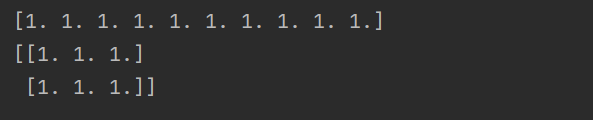### <3>使用ones_like创建与某个数组形状相同的单位数组

ones_like(a（数组）,dtype=float,order=‘C’)

print(np.ones_like(x))#创建一个和x相同形状的单位数组
print(np.ones_like(X))#创建一个和X相同形状的单位数组
print(np.ones_like([2,3]))#创建一个和[2,3]数组形状相同的单位数组
print(np.ones_like([[1,2,3],[4,5,6]]))#创建一个和[[1,2,3],[4,5,6]]数组形状相同的单位数组


运行结果：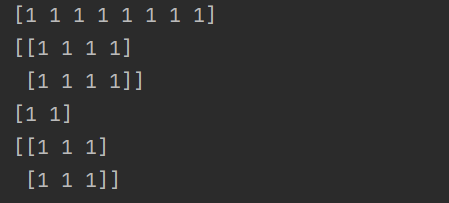### <4>使用zeros创建全是0的数组

np.zeros(shape,dtype=None,order=‘C’)

print(np.zeros(3))#创建一个一行三列的零数组
print(np.zeros((2,4)))#创建一个二行四列的零数组


运行结果：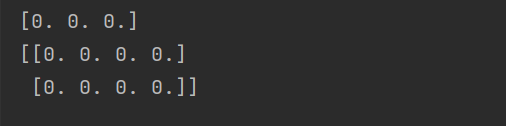### <5>使用zeros_like创建与某个数组形状相同的零数组

np.zeros_like(a(数组)，dtype=None,order=‘C’)

print(np.zeros_like(x))#创建一个和x数组形状相同的零数组
print(np.zeros_like(X))#创建一个和X数组形状相同的零数组
print(np.zeros_like([2,3]))#创建一个和[2,3]数组形状相同的零数组
print(np.zeros_like([[1,2,3],[4,5,6]]))#创建一个和[[1,2,3],[4,5,6]]数组形状相同的零数组


运行结果：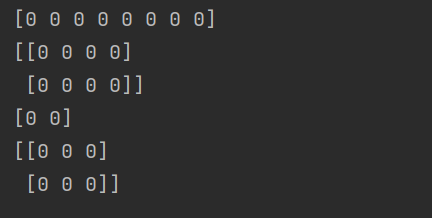### <6>使用empty创建全是0的数组

np.empty(shape,dtype=Nome,order=‘C’)
注意：数据是未初始化的，里面的值可能是随机值，不要用

print(np.empty(5))
print(np.empty((2,3)))


运行结果：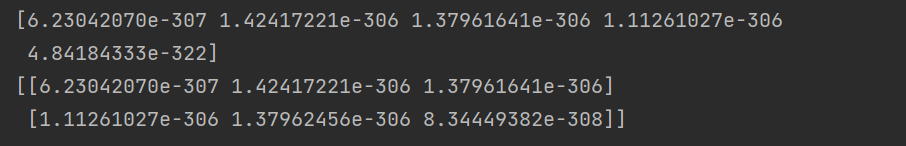### <7>使用empty_like创建形状相同的零数组

np.empty_like(prototype,dtype=None)(也有可能是未初始化数据，为随机值)

print(np.empty_like(x))
print(np.empty_like(X))
print(np.empty_like([2,3]))
print(np.empty_like([[1,2,3],[4,5,6]]))


运行结果：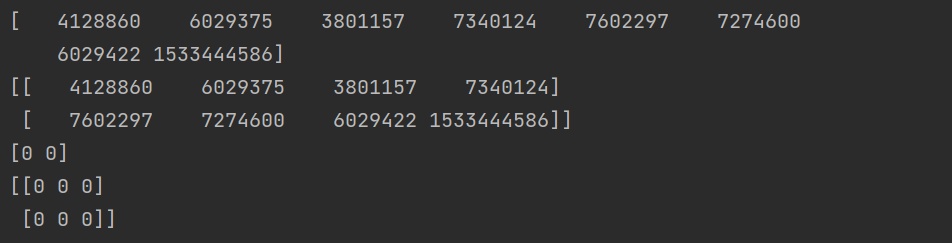### <8>使用full创建指定值的数组

np.full(shape,fill_value,dtype=None,order=‘C’)

print(np.full(4,9))#创建一个一行四列，元素为9的数组
print(np.full((2,3),9))#创建一个二行三列，元素为9的数组



运行结果：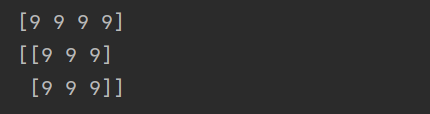### <9>使用full_like创建形状相同的数组

np.full_like(a(数组),fill_value,dtype=None)

print(np.full_like(x,9))#创建一个与x形状相同，元素为9的数组
print(np.full_like(X,9))#创建一个与X形状相同，元素为9的元素
print(np.full_like([2,3,4],9))#创建一个与[2,3,4]形状相同，元素为9的数组
print(np.full_like([[1,2,3],[4,5,6]],9))#创建一个与[[1,2,3],[4,5,6]]形状相同，元素为9的数组


运行结果：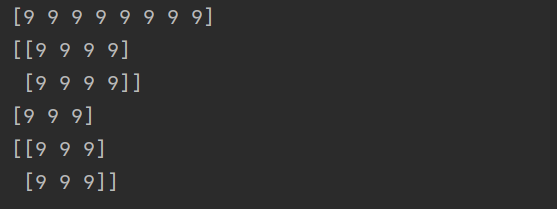### <10>使用random模块生成随机数的数组

np.random.randn(d0,d1,…,dn)

print(np.random.randn())#生成一个随机数
print(np.random.randn(3))#随机生成一个一行三列的数组
print(np.random.randn(2,3))#随机生成一个二行三列的数组
print(np.random.randn(2,3,4))#随机生成一个三维的数组，有两块三行四列的数组组成


运行结果：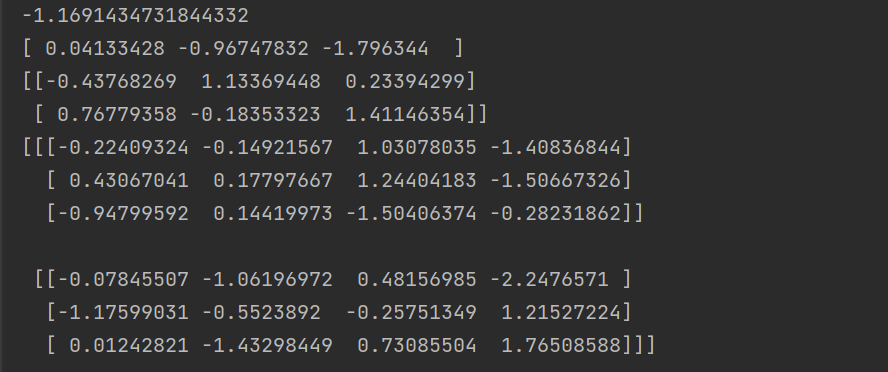## 4.array本身支持的大量操作和函数

A=np.arange(10).reshape(2,5)#创建一个0-10的二行五列的数组
print(A)
print(A.shape)#数组的形状
print(A+1)#对A数组每个元素加一
print(A*2)#对A数组每个元素都乘2
print(np.sin(A))#对A数组每个元素求sin值,还可求其他三角函数值
print(np.exp(A))#对A数组每个元素求ln值
print(np.exp2(A))#对A数组每个元素求log2值


运行结果：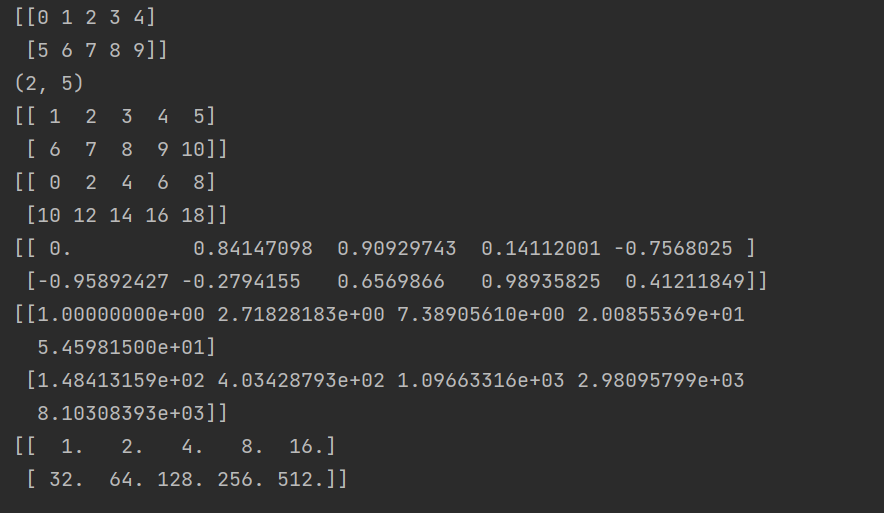B=np.random.randn(2,5)#随机生成一个二行五列的数组
print(A+B)#将数组A和数组B对应元素相加
print(A-B)#将数组A和数组B对应元素相减


运行结果：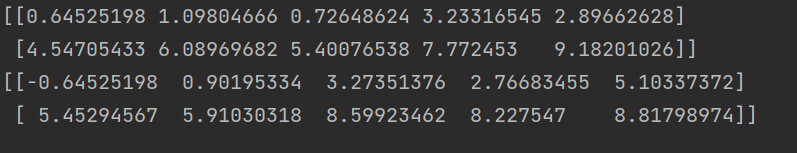展开全文python 开发语言 后端
• ## Numpy 的创建array

千次阅读 2020-12-24 09:07:00
关键字array创建数组dtype：指定数据类型zeros：创建数据全为0ones：创建数据全为1empty：创建数据接近0arrange：按指定范围创建数据linspace：创建线段创建数组a = np.array([2,23,4]) # list 1dprint(a)# [2 23 ...
• 学习Python的童鞋，对numpy都不陌生...如何创建array?可以用tuple也可用list. 其中，dtype用来指定数据类型，int32指，存放int类型最高32位，不过也可以去掉32，直接写int。数据类型float都可以定义数据的长度，也...Python Numpy
• 之前，我们有提到如何下载Numpy并且添加到Pycharm的解释器中，这次，我们来看看numpy中最基础的数组创建。 首先，我们来看看数组的如何创建的 import numpy as np a = np.array([1, 2, 3, 4]) 简简单单，一个数组就...python numpy 数组
• java.lang.reflect.Array类提供了通过静态方法来动态创建和访问Java数组的操作。 1.主要的静态方法 java.lang.reflect.Array提供以下几类静态方法操作： Array.newInstance() ：创建引用类型的数组 Array.set...java反射 数组
• ## JS中常见的Array方法

千次阅读 2020-04-07 21:01:36
​对于JS中的数组(Array)我们经常使用，今天总结了一下常见的Array方法。 1. length 数组长度 let arr = ['a', 'b', 'c', 'd']; console.log(arr.length); 2. join() 以指定的字符将数组连接起来，变成字符串 ...
• ## JS Es6 Array方法

千次阅读 2019-02-21 10:49:32
JS array es6方法 定义数组 const array = [1, 2, 3]; 或者 const array = new Array(); array = ‘1’; 建议尽量使用第一种形式定义数组，采用new的形式在大量的数组定义时，会比较耗时。 new关键字的使用，除了...es6
• Array对象常用的方法 不改变原数组的方法 1、concat() 语法：arrayObject.concat(arrayX,arrayX,......,arrayX)  输入值： 输入值可以是单个元素（多个元素），也可以是单个数组（多个数组） 功能：连接两个或...
• 有5种方法可以创建数组： 从python的其他数据类型 转换 过来，比如 列表list ， 元组tuple 等 numpy 数组创建的 内置函数 ，比如 arange，linspace，ones 等 从磁盘里，或者从其他标准和自定义的格式里 读入...python numpy
• java创建数组的两种方法 1，静态初始化 int[] array={1,2,3,4,5}; 2，动态初始化 1）先分配空间再给数组元素赋值 int[] array=new int; //需指定数组中的元素个数 for (int i = 0; i < array.length; i++) {...java 数组
• 方法1:for循环 for循环其实是标准的C风格语法。 let someArray = [1, "string", false]; ​ for (var i = 0; i &lt; someArray.length; i ++) { ​  console.log(someArray[i]); // 1, "...遍历数组
• numpy可以快速的实现多维度的矩阵计算，本文介绍python中使用numpy.array函数创建数组的有关内容。1、numpy.array函数是由N个非负整数组成的tuple(元组)，用于指定每个维度的大小。数组中项目的类型由单独的data-...
• ## js Array 原生方法

千次阅读 2016-09-18 22:14:00数组
• 它的计算速度非常快所以经常被用来做底层的数据运算，numpy运算的所用的数据结构叫做ndarray，也叫做数组（array），那么我们怎么创建一个数组，也就是对数组进行初始化呢，这里就要用到np.array()这个方法，...
• ## C++ array使用方法详细介绍

万次阅读 多人点赞 2019-11-04 11:20:36
由于array创建的时候必须明确指定大小，而且array为固定容器，因此max_size()与size()的返回值相同。 通常使用 size() 函数。 返回值类型为size_t 即无符号整数 使用size()可以有效避免使用for遍历数组时...c++
• 问题乍看之下，创建全0数组应该是一件不能再简单的事情了：var arr = [0,0,0,0,0,0];...所以今天创建全0数组的时候，我用了以下方法：var arr = new Array(10);//这里等同于//var arr = [];arr.length = 10;arr ...
• 1、创建Array对象 Array用于创建数组对象及对数组进行处理操作。 创建Array对象的方式有三种： var arr1 = new Array(); //创建空数组 var arr2 = new Array(4); //创建4个长度的数组 var arr3 = new Array(1,2,3,5)...
• ## JavaScript数组Array常用方法汇总

千次阅读 多人点赞 2021-10-31 15:24:19
一.JavaScript中创建数组的方式 （1）使用 Array 构造函数： var arr1 = new Array(); //创建一个空数组 var arr2 = new Array(20); // 创建一个包含20项的数组 var arr3 = new Array("lily","lucy","Tom"); // ...
• TIA博途中数组Array的基本使用方法详解 ARRAY类型是由数目固定且数据类型相同的元素组成的数据结构。 ARRAY类型的定义和使用需要注意以下几点： • ARRAY类型可以在DB、OB/FC/FB接口区、PLC数据类型处定义；无法在...数组 数组寻址
• 前两天在刷leetcode时，遇到一个设计题，需要设计一个哈希集合，当时的想法就是创建一个链表数组，用链表来解决哈希冲突问题。 然而在编写时，编译器报错，如下图所示。 分析 为什么会出现编译错误呢？这里就是泛型...java 泛型 泛型数组
• ## Kotlin - 数组 Array

千次阅读 2021-12-10 23:40:46
创建方式 Array arrayOfXXX () arrayOf () val array1:Array<String> = arrayOf("你", "我", "他") val array2:Array<Person> = arrayOf(person1, person2) ...kotlin
• 需求 1、参数是一个数组的个数，比如 7 2、希望得到的结果如下 [{ label: ‘1’, value: 1 }, { label: ‘2’, ...Array.from({ length: 7}, ( k, v ) => ({ label: v+1, value: v+1 }))
• 各种版本的操作系统安装方法不一。 官网：https://pypi.org/project/numpy/#files 我使用的是pycharm+Anaconda，anaconda是python中管理包的工具，在我们安装好anaconda的时候，会自带很多python的包。当我们在...
• ## Scala之Array的方法

万次阅读 多人点赞 2018-08-05 11:06:14
Scala：Array（集合、序列） Scala开篇（目录） 数组是一种可变的、可索引的数据集合。在Scala中用Array[T]的形式来表示Java中的数组形式 T[]。 val numbers = Array(1, 2, 3, 4) //声明一个数组对象 val first =...
• Array.from() 作用：从一个类似数组或可迭代对象中创建一个新的，浅拷贝的数组实例。 （1）：这里讲一下深拷贝和浅拷贝的区别 浅拷贝：只复制指向某个对象的指针，而不复制对象本身，新旧对象还是共享同一块内存。 ...
• Scala：Array（集合、序列） Scala开篇（目录） 数组是一种可变的、可索引的数据集合。在Scala中用Array[T]的形式来表示Java中的数组形式 T[]。 val numbers = Array(1, 2, 3, 4) //声明一个数组对象 val first = ...scala
• Pandas中把dataframe转成array方法使用df=df.values，可以把Pandas中的dataframe转成numpy中的array以上这篇Pandas中把dataframe转成array方法就是小编分享给大家的全部内容了，希望能给大家一个参考，也希望......